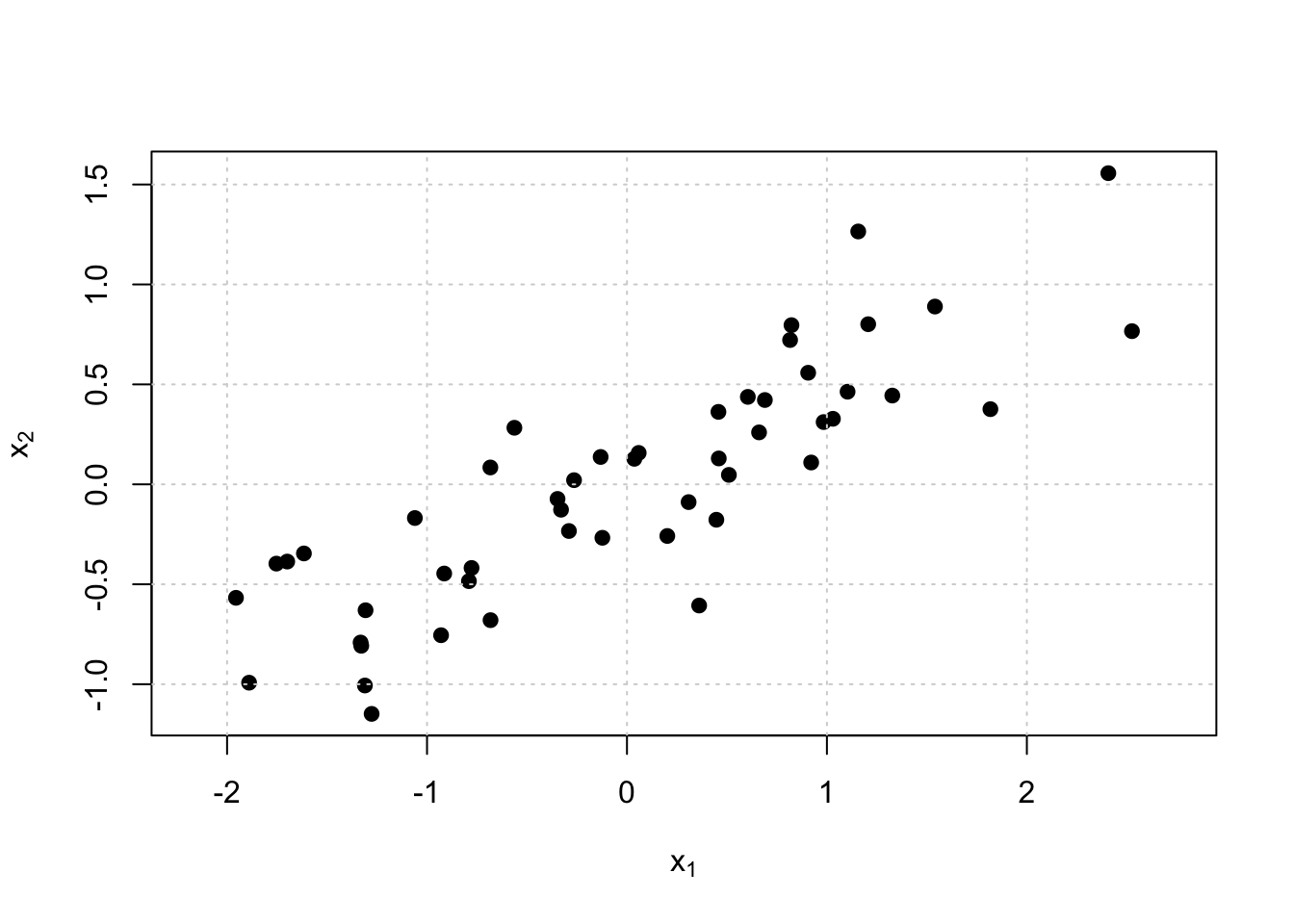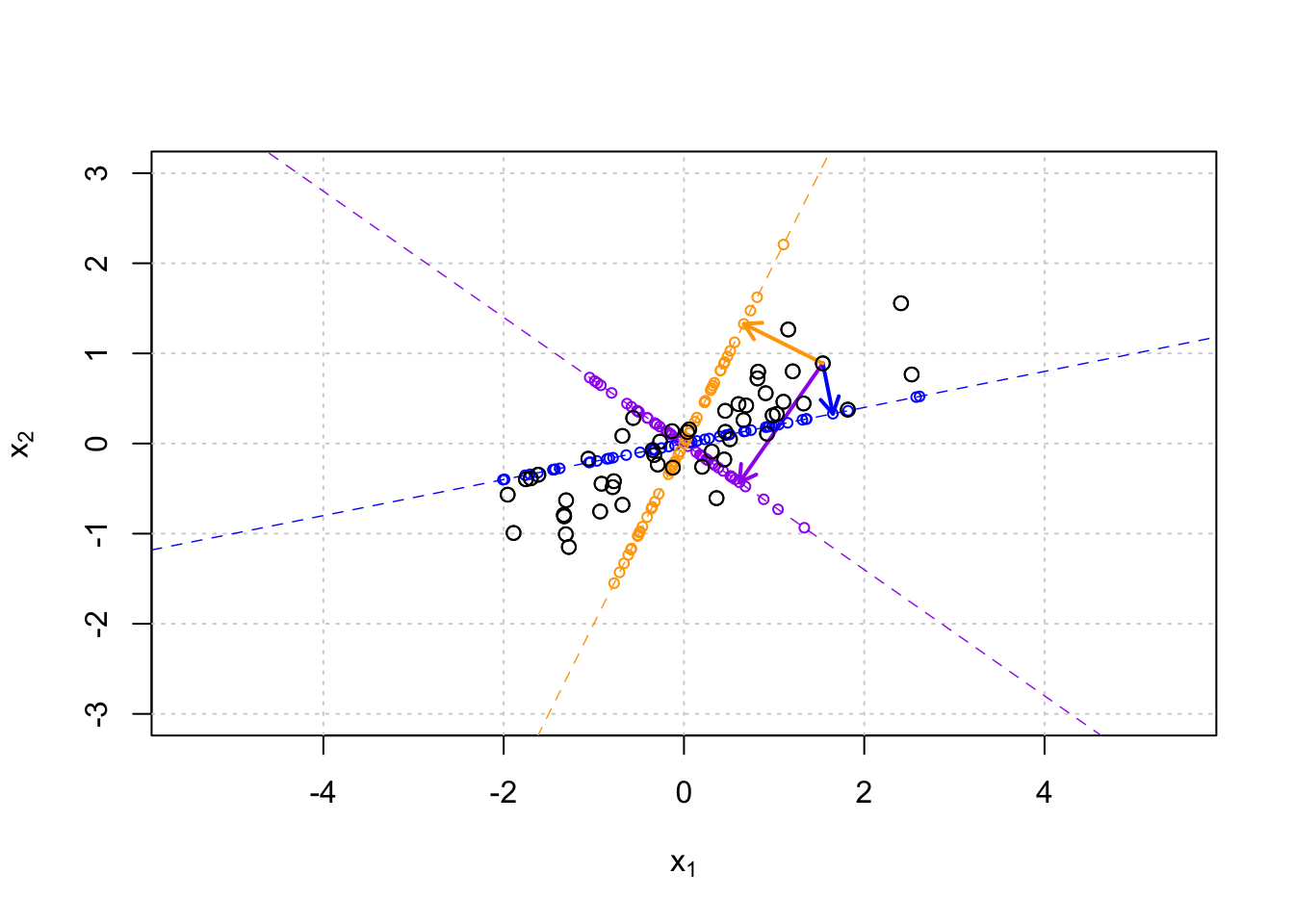Now we move from variable selection to dimension reduction. The goal is to represent high dimensional data in a useful way in a low dimensional subspace, for example, to visualize the data in human interpretable ways or to pre-process the data for downstream analyses that benefit from reduced data size. The key question is what is a useful way to reduce dimension. Intuitively, given a target dimension, we want to find a mapping from the original data to the target space by keeping as much of the original information as possible. PCA (first defined in 1901 by K. Pearson) is one of the most widely used method for this purpose, and one with a clear statistical motivation and linear algebraic implementation, so we will start from it.

Example in 2 dimensions

Let’s consider a set of n=50 points on 2D space (p=2):

set.seed(18)
n = 50
x.1 = rnorm(n)
x.2 = 0.4*x.1 + rnorm(n, 0, 0.4)
X = cbind(x.1 - mean(x.1), x.2 - mean(x.2)) #Always mean-center for PCA
plot(X, asp = 1, pch = 19, xlab = expression(x), ylab = expression(x))
grid()var(X) #what is variance in x and y direction? and what is the covariance?
##           [,1]      [,2]
## [1,] 1.2526074 0.5590945
## [2,] 0.5590945 0.3478777

The variables are quite correlated, so they are telling partly a redundant story. Do we really need 2 dimensions to represent these data? Or could 1 dimension be enough? How could we put these points to one dimension in a useful way that would preserve main properties of their relationships?

Let’s draw some lines through the origin and project the data on those lines. (Since data are mean centered, the origin is at the center of the data set.) Then we will have 1-dimensional representations of the data. The unit vector of a line that passes through the origin with slope $$b$$ is $$\pmb u = (1,b)^T/\sqrt{1+b^2}$$. The projection of a point $$\pmb x=(x_1,x_2)^T$$ on that line is $(\pmb u^T \pmb x) \pmb u = \left(\frac{(1,b)\cdot(x_1,x_2)}{\sqrt{1+b^2}}\right)\frac{(1,b)^T}{\sqrt{1+b^2}}= \left(\frac{x_1+b x_2}{1+b^2}\right) (1,b)^T$ And the coordinate of the point along the 1-dimensional line is $(\pmb u^T \pmb x) = \left(\frac{x_1+b x_2}{\sqrt{1+b^2}}\right).$

plot(X, pch = 1, xlim = c(-3,3), ylim = c(-3,3), asp = 1,
xlab = expression(x), ylab = expression(x))
grid()
b = c(-0.7, 0.2, 2) #example lines
cols = c("purple", "blue", "orange")
vars = rep(NA, length(b)) #variances of the projected point on each line
for(ii in 1:length(b)){
abline(0, b[ii], col = cols[ii], lty = 2, lwd = 0.7)
coord.on.line = (X[,1] + X[,2]*b[ii]) / sqrt(1 + b[ii]^2) #coordinate along each line
z = coord.on.line/sqrt(1 + b[ii]^2) #temp variable
points(z, z*b[ii], col = cols[ii], pch = 1, cex = 0.7) #points on the line
#Show projection for point jj=10 using arrows from point to lines
jj = 10
arrows(X[jj,1], X[jj,2], z[jj], z[jj]*b[ii], code = 2,
length = 0.1, lwd = 2, col = cols[ii])
vars[ii] = var(coord.on.line)
}
points(X, pch = 1) #redraw the original points on topcbind(b, vars) #see what is the variance of the projected points on each line
##         b      vars
## [1,] -0.7 0.4297552
## [2,]  0.2 1.4328465
## [3,]  2.0 0.9760993

Because the projections are orthogonal, we can think that the projections preserve (some) of the original relationships between the points. It seems that line 2 (with slope 0.2) separates the points most from each other as on that line the projected points have the largest empirical variance. In that sense it preserves more information about the original data than the other two lines. Think about the two extremes that would be the useless case where each original point is projected on the same point on a line, whence we lose all the information about the differences between the original data units, and the case of perfect information where the original data was already on a line and therefore we do not lose anything by simply recording the coordinates on that line. In reality we always work with data in between these extremes, and in PCA the criteria of finding the subspace is to choose the line on which the projections preserve the highest possible variance.

What would be the line on which the points had the largest variance? Let’s find the unit vector $$\pmb u = (u_1,u_2)^T$$ $$(u_1^2 + u_2^2 =1)$$ that maximizes the empirical variance of the projections of $$X$$ on that line. As the columns of $$\pmb X$$ are mean-centered then also the mean of $$\pmb X \pmb u$$ is 0, and variance is proportional to the sum of squares of $$\pmb X \pmb u$$: $\widehat{\textrm{Var}}\left(\pmb X \pmb u \right) \propto \pmb u^T \pmb X^T \pmb X \pmb u.$

We can use the method of Lagrange multipliers to maximize this quantity among all unit vectors $$\pmb u$$ (i.e. when $$\pmb u^T \pmb u - 1 = 0$$). This method requires us to maximize the extended function $$f(\pmb u, \alpha) = \pmb u^T \pmb X^T \pmb X \pmb u + \alpha (\pmb u^T \pmb u - 1)$$ with respect to $$\pmb u$$ and $$\alpha$$. Derivative with respect to $$\pmb u$$ is $\frac{\partial f(\pmb u, \alpha)}{\partial \pmb u} = 2\pmb X^T \pmb X \pmb u + 2\alpha \pmb u,$ and equals to zero when $$\pmb X^T \pmb X \pmb u = -\alpha \pmb u,$$ that is, when $$\pmb u$$ is an eigenvector of matrix $$\pmb X^T \pmb X$$ and $$-\alpha$$ is the corresponding eigenvalue. Among all eigenvectors, we thus choose the one that maximizes $$\pmb u^T \pmb X^T \pmb X \pmb u = -\alpha \pmb u^T\pmb u = -\alpha,$$ which shows that $$\pmb u$$ should correspond to the largest eigenvalue of $$\pmb X^T \pmb X$$.

Let’s apply this result in practice.

eig = eigen(t(X) %*% X)
eig$values ##  74.451730 3.972042 eig$vectors #are in columns, in decreasing order of eigenvalues
##            [,1]       [,2]
## [1,] -0.9024967  0.4306969
## [2,] -0.4306969 -0.9024967
u = eig\$vectors[,1] #corresponds to the largest eigenvalue.
plot(X, pch = 1, xlim = c(-3,3), ylim = c(-3,3), asp = 1,
xlab = expression(x), ylab = expression(x) ) #plot the data again
abline(0, u/u, lty = 2, col = "red") #add max variance line whose unit vector is u
#do projections on the max variance line
coord.on.line = (X %*% u) #coordinates of points in X projected on line u
points( u*coord.on.line, u*coord.on.line, col = "red", cex = 0.7)